# Mean State

Period Mean (original grids) [Watt m-2]
Model Period Mean (intersection) [Watt m-2]
Model Period Mean (complement) [Watt m-2]
Benchmark Period Mean (intersection) [Watt m-2]
Benchmark Period Mean (complement) [Watt m-2]
Bias [Watt m-2]
RMSE [Watt m-2]
Phase Shift [months]
Bias Score 
RMSE Score 
Seasonal Cycle Score 
Spatial Distribution Score 
Interannual Variability Score 
Overall Score 
Benchmark [-] 179.
CRUNCEPv7 [-] 192. 192. 177. 216. 14.5 24.4 1.07 0.429 0.451 0.858 0.933 0.511 0.606
GSWP3v1 [-] 183. 183. 177. 216. 5.93 16.8 0.514 0.620 0.524 0.935 0.921 0.727 0.709
WFDEI [-] 168. 167. 177. 216. -9.51 24.0 1.07 0.502 0.434 0.845 0.947 0.692 0.642
Period Mean (original grids) [Watt m-2]
Model Period Mean (intersection) [Watt m-2]
Model Period Mean (complement) [Watt m-2]
Benchmark Period Mean (intersection) [Watt m-2]
Benchmark Period Mean (complement) [Watt m-2]
Bias [Watt m-2]
RMSE [Watt m-2]
Phase Shift [months]
Bias Score 
RMSE Score 
Seasonal Cycle Score 
Spatial Distribution Score 
Interannual Variability Score 
Overall Score 
Benchmark [-] 189.
CRUNCEPv7 [-] 198. 198. 188. 224. 9.44 22.6 1.61 0.570 0.465 0.761 0.888 0.638 0.631
GSWP3v1 [-] 195. 195. 188. 224. 6.39 16.2 0.423 0.672 0.536 0.965 0.999 0.767 0.746
WFDEI [-] 190. 190. 188. 224. 2.13 19.5 0.534 0.613 0.504 0.940 0.997 0.737 0.716
Period Mean (original grids) [Watt m-2]
Model Period Mean (intersection) [Watt m-2]
Model Period Mean (complement) [Watt m-2]
Benchmark Period Mean (intersection) [Watt m-2]
Benchmark Period Mean (complement) [Watt m-2]
Bias [Watt m-2]
RMSE [Watt m-2]
Phase Shift [months]
Bias Score 
RMSE Score 
Seasonal Cycle Score 
Spatial Distribution Score 
Interannual Variability Score 
Overall Score 
Benchmark [-] 194.
CRUNCEPv7 [-] 195. 195. 192. 221. 2.95 22.4 1.07 0.693 0.503 0.861 0.735 0.528 0.637
GSWP3v1 [-] 197. 197. 192. 221. 4.44 18.6 0.496 0.707 0.567 0.932 0.960 0.665 0.733
WFDEI [-] 196. 196. 192. 221. 3.85 22.6 0.807 0.691 0.495 0.905 0.998 0.633 0.703
Period Mean (original grids) [Watt m-2]
Model Period Mean (intersection) [Watt m-2]
Model Period Mean (complement) [Watt m-2]
Benchmark Period Mean (intersection) [Watt m-2]
Benchmark Period Mean (complement) [Watt m-2]
Bias [Watt m-2]
RMSE [Watt m-2]
Phase Shift [months]
Bias Score 
RMSE Score 
Seasonal Cycle Score 
Spatial Distribution Score 
Interannual Variability Score 
Overall Score 
Benchmark [-] 81.3
CRUNCEPv7 [-] 72.0 72.1 80.9 86.2 -10.0 23.5 0.275 0.861 0.719 0.982 0.940 0.639 0.810
GSWP3v1 [-] 69.8 69.9 80.9 86.2 -11.6 21.8 0.200 0.843 0.740 0.987 0.945 0.720 0.829
WFDEI [-] 60.1 60.3 80.9 86.2 -21.0 29.0 0.271 0.736 0.715 0.982 0.916 0.788 0.808
Period Mean (original grids) [Watt m-2]
Model Period Mean (intersection) [Watt m-2]
Model Period Mean (complement) [Watt m-2]
Benchmark Period Mean (intersection) [Watt m-2]
Benchmark Period Mean (complement) [Watt m-2]
Bias [Watt m-2]
RMSE [Watt m-2]
Phase Shift [months]
Bias Score 
RMSE Score 
Seasonal Cycle Score 
Spatial Distribution Score 
Interannual Variability Score 
Overall Score 
Benchmark [-] 189.
CRUNCEPv7 [-] 187. 187. 183. 210. 6.23 26.2 1.53 0.481 0.411 0.774 0.548 0.466 0.515
GSWP3v1 [-] 178. 178. 183. 210. -1.23 20.6 0.686 0.600 0.455 0.906 0.730 0.715 0.643
WFDEI [-] 165. 165. 183. 210. -14.0 27.6 1.32 0.502 0.406 0.806 0.923 0.576 0.603
Period Mean (original grids) [Watt m-2]
Model Period Mean (intersection) [Watt m-2]
Model Period Mean (complement) [Watt m-2]
Benchmark Period Mean (intersection) [Watt m-2]
Benchmark Period Mean (complement) [Watt m-2]
Bias [Watt m-2]
RMSE [Watt m-2]
Phase Shift [months]
Bias Score 
RMSE Score 
Seasonal Cycle Score 
Spatial Distribution Score 
Interannual Variability Score 
Overall Score 
Benchmark [-] 105.
CRUNCEPv7 [-] 109. 109. 104. 127. 4.44 20.7 0.171 0.899 0.761 0.989 0.978 0.599 0.831
GSWP3v1 [-] 102. 102. 104. 127. -2.18 14.9 0.0819 0.947 0.812 0.995 0.998 0.630 0.866
WFDEI [-] 94.5 94.5 104. 127. -9.36 20.8 0.202 0.873 0.770 0.986 0.958 0.736 0.849
Period Mean (original grids) [Watt m-2]
Model Period Mean (intersection) [Watt m-2]
Model Period Mean (complement) [Watt m-2]
Benchmark Period Mean (intersection) [Watt m-2]
Benchmark Period Mean (complement) [Watt m-2]
Bias [Watt m-2]
RMSE [Watt m-2]
Phase Shift [months]
Bias Score 
RMSE Score 
Seasonal Cycle Score 
Spatial Distribution Score 
Interannual Variability Score 
Overall Score 
Benchmark [-] 140.
CRUNCEPv7 [-] 149. 149. 139. 154. 9.39 26.6 0.561 0.743 0.691 0.956 0.969 0.643 0.782
GSWP3v1 [-] 136. 136. 139. 154. -3.31 17.0 0.232 0.890 0.743 0.981 0.998 0.628 0.831
WFDEI [-] 142. 141. 139. 154. 1.92 24.5 0.553 0.825 0.657 0.952 0.964 0.722 0.796
Period Mean (original grids) [Watt m-2]
Model Period Mean (intersection) [Watt m-2]
Model Period Mean (complement) [Watt m-2]
Benchmark Period Mean (intersection) [Watt m-2]
Benchmark Period Mean (complement) [Watt m-2]
Bias [Watt m-2]
RMSE [Watt m-2]
Phase Shift [months]
Bias Score 
RMSE Score 
Seasonal Cycle Score 
Spatial Distribution Score 
Interannual Variability Score 
Overall Score 
Benchmark [-] 180.
CRUNCEPv7 [-] 195. 195. 178. 210. 16.4 31.7 0.424 0.528 0.539 0.970 0.965 0.532 0.679
GSWP3v1 [-] 175. 175. 178. 210. -1.06 18.7 0.290 0.800 0.620 0.980 0.929 0.635 0.764
WFDEI [-] 175. 175. 178. 210. -2.52 25.9 0.402 0.689 0.546 0.970 0.998 0.626 0.729
Period Mean (original grids) [Watt m-2]
Model Period Mean (intersection) [Watt m-2]
Model Period Mean (complement) [Watt m-2]
Benchmark Period Mean (intersection) [Watt m-2]
Benchmark Period Mean (complement) [Watt m-2]
Bias [Watt m-2]
RMSE [Watt m-2]
Phase Shift [months]
Bias Score 
RMSE Score 
Seasonal Cycle Score 
Spatial Distribution Score 
Interannual Variability Score 
Overall Score 
Benchmark [-] 74.6
CRUNCEPv7 [-] 63.7 64.1 74.6 74.8 -11.5 22.8 0.244 0.833 0.701 0.984 0.867 0.646 0.789
GSWP3v1 [-] 60.5 60.9 74.6 74.8 -14.4 20.3 0.228 0.801 0.735 0.985 0.911 0.679 0.808
WFDEI [-] 50.8 51.3 74.6 74.8 -24.0 28.6 0.237 0.693 0.708 0.984 0.939 0.763 0.799
Period Mean (original grids) [Watt m-2]
Model Period Mean (intersection) [Watt m-2]
Model Period Mean (complement) [Watt m-2]
Benchmark Period Mean (intersection) [Watt m-2]
Benchmark Period Mean (complement) [Watt m-2]
Bias [Watt m-2]
RMSE [Watt m-2]
Phase Shift [months]
Bias Score 
RMSE Score 
Seasonal Cycle Score 
Spatial Distribution Score 
Interannual Variability Score 
Overall Score 
Benchmark [-] 193.
CRUNCEPv7 [-] 193. 193. 193. 202. -1.42 20.6 0.505 0.825 0.679 0.965 0.942 0.582 0.779
GSWP3v1 [-] 190. 190. 193. 202. -3.35 18.2 0.157 0.850 0.706 0.988 0.998 0.606 0.809
WFDEI [-] 194. 194. 193. 202. -0.299 23.6 0.286 0.831 0.629 0.979 0.991 0.690 0.791
Period Mean (original grids) [Watt m-2]
Model Period Mean (intersection) [Watt m-2]
Model Period Mean (complement) [Watt m-2]
Benchmark Period Mean (intersection) [Watt m-2]
Benchmark Period Mean (complement) [Watt m-2]
Bias [Watt m-2]
RMSE [Watt m-2]
Phase Shift [months]
Bias Score 
RMSE Score 
Seasonal Cycle Score 
Spatial Distribution Score 
Interannual Variability Score 
Overall Score 
Benchmark [-] 120.
CRUNCEPv7 [-] 132. 132. 117. 151. 15.4 28.2 0.236 0.736 0.746 0.984 0.994 0.643 0.808
GSWP3v1 [-] 113. 113. 117. 151. -1.04 16.2 0.267 0.906 0.803 0.981 0.998 0.620 0.852
WFDEI [-] 113. 113. 117. 151. -1.91 23.0 0.292 0.866 0.734 0.979 0.974 0.741 0.838
Period Mean (original grids) [Watt m-2]
Model Period Mean (intersection) [Watt m-2]
Model Period Mean (complement) [Watt m-2]
Benchmark Period Mean (intersection) [Watt m-2]
Benchmark Period Mean (complement) [Watt m-2]
Bias [Watt m-2]
RMSE [Watt m-2]
Phase Shift [months]
Bias Score 
RMSE Score 
Seasonal Cycle Score 
Spatial Distribution Score 
Interannual Variability Score 
Overall Score 
Benchmark [-] 168.
CRUNCEPv7 [-] 143. 143. 143. 180. 0.159 24.0 0.587 0.710 0.631 0.935 0.954 0.552 0.736
GSWP3v1 [-] 137. 137. 143. 180. -5.71 18.9 0.261 0.786 0.673 0.974 0.966 0.623 0.783
WFDEI [-] 136. 136. 143. 180. -6.95 24.3 0.483 0.723 0.619 0.950 0.959 0.655 0.754
Period Mean (original grids) [Watt m-2]
Model Period Mean (intersection) [Watt m-2]
Model Period Mean (complement) [Watt m-2]
Benchmark Period Mean (intersection) [Watt m-2]
Benchmark Period Mean (complement) [Watt m-2]
Bias [Watt m-2]
RMSE [Watt m-2]
Phase Shift [months]
Bias Score 
RMSE Score 
Seasonal Cycle Score 
Spatial Distribution Score 
Interannual Variability Score 
Overall Score 
Benchmark [-] 175.
CRUNCEPv7 [-] 168. 168. 173. 224. -4.36 21.7 0.385 0.706 0.696 0.967 0.996 0.590 0.775
GSWP3v1 [-] 162. 162. 173. 224. -10.2 19.6 0.295 0.716 0.729 0.974 0.990 0.578 0.786
WFDEI [-] 172. 172. 173. 224. -0.873 20.7 0.465 0.741 0.690 0.961 0.994 0.561 0.773
Period Mean (original grids) [Watt m-2]
Model Period Mean (intersection) [Watt m-2]
Model Period Mean (complement) [Watt m-2]
Benchmark Period Mean (intersection) [Watt m-2]
Benchmark Period Mean (complement) [Watt m-2]
Bias [Watt m-2]
RMSE [Watt m-2]
Phase Shift [months]
Bias Score 
RMSE Score 
Seasonal Cycle Score 
Spatial Distribution Score 
Interannual Variability Score 
Overall Score 
Benchmark [-] 80.0
CRUNCEPv7 [-] 74.2 74.0 79.8 82.9 -7.97 22.3 0.115 0.858 0.726 0.992 0.814 0.631 0.791
GSWP3v1 [-] 69.1 69.0 79.8 82.9 -12.2 20.7 0.0842 0.827 0.753 0.994 0.910 0.736 0.829
WFDEI [-] 62.1 62.0 79.8 82.9 -19.3 28.1 0.176 0.748 0.712 0.988 0.853 0.770 0.797
Period Mean (original grids) [Watt m-2]
Model Period Mean (intersection) [Watt m-2]
Model Period Mean (complement) [Watt m-2]
Benchmark Period Mean (intersection) [Watt m-2]
Benchmark Period Mean (complement) [Watt m-2]
Bias [Watt m-2]
RMSE [Watt m-2]
Phase Shift [months]
Bias Score 
RMSE Score 
Seasonal Cycle Score 
Spatial Distribution Score 
Interannual Variability Score 
Overall Score 
Benchmark [-] 205.
CRUNCEPv7 [-] 189. 190. 200. 227. -8.91 28.6 0.818 0.608 0.590 0.916 0.661 0.524 0.648
GSWP3v1 [-] 192. 192. 200. 227. -6.02 20.0 0.221 0.770 0.638 0.977 0.896 0.625 0.757
WFDEI [-] 193. 194. 200. 227. -6.84 25.3 0.303 0.692 0.599 0.969 0.963 0.601 0.737
Period Mean (original grids) [Watt m-2]
Model Period Mean (intersection) [Watt m-2]
Model Period Mean (complement) [Watt m-2]
Benchmark Period Mean (intersection) [Watt m-2]
Benchmark Period Mean (complement) [Watt m-2]
Bias [Watt m-2]
RMSE [Watt m-2]
Phase Shift [months]
Bias Score 
RMSE Score 
Seasonal Cycle Score 
Spatial Distribution Score 
Interannual Variability Score 
Overall Score 
Benchmark [-] 153.
CRUNCEPv7 [-] 157. 157. 151. 194. 6.76 19.6 0.445 0.845 0.744 0.971 0.999 0.546 0.808
GSWP3v1 [-] 144. 144. 151. 194. -4.68 15.8 0.124 0.913 0.772 0.992 0.999 0.618 0.844
WFDEI [-] 150. 150. 151. 194. 0.713 22.8 0.281 0.908 0.679 0.980 0.992 0.755 0.832
Period Mean (original grids) [Watt m-2]
Model Period Mean (intersection) [Watt m-2]
Model Period Mean (complement) [Watt m-2]
Benchmark Period Mean (intersection) [Watt m-2]
Benchmark Period Mean (complement) [Watt m-2]
Bias [Watt m-2]
RMSE [Watt m-2]
Phase Shift [months]
Bias Score 
RMSE Score 
Seasonal Cycle Score 
Spatial Distribution Score 
Interannual Variability Score 
Overall Score 
Benchmark [-] 115.
CRUNCEPv7 [-] 123. 123. 114. 123. 8.11 20.5 0.210 0.869 0.778 0.986 0.970 0.578 0.827
GSWP3v1 [-] 112. 112. 114. 123. -2.84 14.6 0.0871 0.926 0.826 0.994 0.999 0.637 0.868
WFDEI [-] 111. 111. 114. 123. -3.11 19.9 0.148 0.913 0.767 0.990 0.937 0.790 0.861
Period Mean (original grids) [Watt m-2]
Model Period Mean (intersection) [Watt m-2]
Model Period Mean (complement) [Watt m-2]
Benchmark Period Mean (intersection) [Watt m-2]
Benchmark Period Mean (complement) [Watt m-2]
Bias [Watt m-2]
RMSE [Watt m-2]
Phase Shift [months]
Bias Score 
RMSE Score 
Seasonal Cycle Score 
Spatial Distribution Score 
Interannual Variability Score 
Overall Score 
Benchmark [-] 162.
CRUNCEPv7 [-] 156. 156. 162. 171. -5.22 17.5 0.262 0.903 0.792 0.982 0.985 0.613 0.844
GSWP3v1 [-] 160. 160. 162. 171. -1.80 14.7 0.134 0.934 0.813 0.991 1.00 0.649 0.867
WFDEI [-] 169. 169. 162. 171. 7.04 23.3 0.259 0.884 0.729 0.983 0.980 0.805 0.852
Period Mean (original grids) [Watt m-2]
Model Period Mean (intersection) [Watt m-2]
Model Period Mean (complement) [Watt m-2]
Benchmark Period Mean (intersection) [Watt m-2]
Benchmark Period Mean (complement) [Watt m-2]
Bias [Watt m-2]
RMSE [Watt m-2]
Phase Shift [months]
Bias Score 
RMSE Score 
Seasonal Cycle Score 
Spatial Distribution Score 
Interannual Variability Score 
Overall Score 
Benchmark [-] 176.
CRUNCEPv7 [-] 183. 183. 174. 226. 9.20 22.7 1.44 0.623 0.431 0.791 0.796 0.476 0.592
GSWP3v1 [-] 174. 174. 174. 226. 0.816 17.1 0.475 0.829 0.468 0.940 0.948 0.646 0.716
WFDEI [-] 160. 160. 174. 226. -14.4 26.8 1.44 0.495 0.405 0.805 0.998 0.533 0.607
Period Mean (original grids) [Watt m-2]
Model Period Mean (intersection) [Watt m-2]
Model Period Mean (complement) [Watt m-2]
Benchmark Period Mean (intersection) [Watt m-2]
Benchmark Period Mean (complement) [Watt m-2]
Bias [Watt m-2]
RMSE [Watt m-2]
Phase Shift [months]
Bias Score 
RMSE Score 
Seasonal Cycle Score 
Spatial Distribution Score 
Interannual Variability Score 
Overall Score 
Benchmark [-] 182.
CRUNCEPv7 [-] 185. 185. 181. 201. 3.85 23.1 0.998 0.788 0.533 0.877 0.909 0.467 0.684
GSWP3v1 [-] 181. 182. 181. 201. 0.0675 19.0 0.293 0.888 0.574 0.965 0.999 0.613 0.769
WFDEI [-] 175. 175. 181. 201. -6.25 24.9 0.569 0.772 0.506 0.942 0.998 0.675 0.733
Period Mean (original grids) [Watt m-2]
Model Period Mean (intersection) [Watt m-2]
Model Period Mean (complement) [Watt m-2]
Benchmark Period Mean (intersection) [Watt m-2]
Benchmark Period Mean (complement) [Watt m-2]
Bias [Watt m-2]
RMSE [Watt m-2]
Phase Shift [months]
Bias Score 
RMSE Score 
Seasonal Cycle Score 
Spatial Distribution Score 
Interannual Variability Score 
Overall Score 
Benchmark [-] 176.
CRUNCEPv7 [-] 177. 176. 176. 178. -1.34 28.1 0.536 0.721 0.664 0.950 0.815 0.510 0.721
GSWP3v1 [-] 178. 176. 176. 178. -2.72 20.8 0.309 0.840 0.697 0.970 0.998 0.605 0.801
WFDEI [-] 181. 181. 176. 178. 1.90 28.3 0.698 0.753 0.647 0.923 0.979 0.615 0.761

# Temporally integrated period mean

BENCHMARK MEAN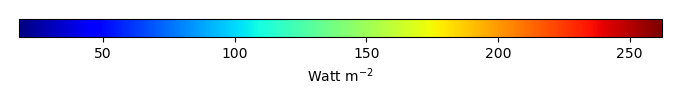MODEL MEANBIAS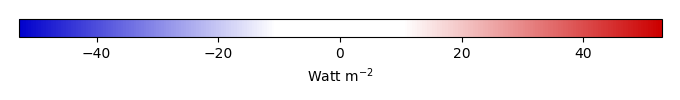BIAS SCORERMSE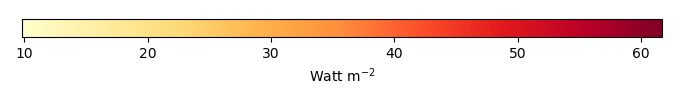RMSE SCOREBENCHMARK INTERANNUAL VARIABILITY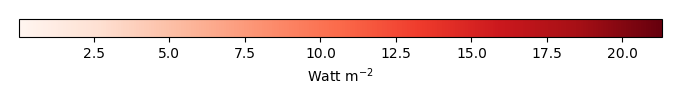MODEL INTERANNUAL VARIABILITYINTERANNUAL VARIABILITY SCOREBENCHMARK MAX MONTHMODEL MAX MONTHDIFFERENCE IN MAX MONTH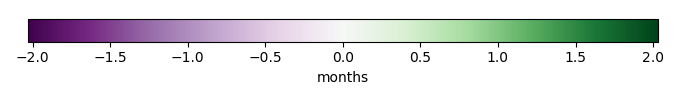SEASONAL CYCLE SCORESPATIAL TAYLOR DIAGRAMMODEL COLORS# Spatially integrated regional mean

MODEL COLORSREGIONAL MEANANNUAL CYCLEMONTHLY ANOMALYANNUAL CYCLE# All Models

BenchmarkCRUNCEPv7GSWP3v1WFDEI# Data Information

creation_date: Tue Jul 1 08:29:23 PDT 2014

source_file: This product is generated from monthly 1 degree GEWEX SRB Radiation observations